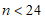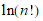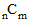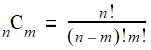# NMath User's Guide

39.1 Combinatorial Functions (.NET, C#, CSharp, VB, Visual Basic, F#)

The static Factorial() method on class StatsFunctions returns n!, the number of ways that n objects can be permuted. A lookup table is used forfor faster access. For example:

Code Example – C# factorial

```int i = StatsFunctions.Factorial( 20 );
```
```// i = 2,432,902,008,176,640,000
```

FactorialLn() returns the natural log factorial of n,.

The static Binomial() method returns the binomial coefficient. The binomial coefficient("choose m") is the number of ways of picking m unordered outcomes from n possibilities:For instance:

Code Example – C# binomial

```int nCm = StatsFunctions.Binomial( 6, 4 );
```

BinomialLn() returns the natural log of the binomial coefficient.

Top

Top